•• Boltzmann Constant

The Boltzmann Constant (Boltzmann’s Constant) is a fundamental physics constant that plays a pivotal role in almost every single statistical formulation in both quantum and classical physics. It is named after Austrian physicist, Ludwig Boltzmann (1844 – 1906), who made significant contributions to the foundation and development of a particular branch of theoretical physics known as statistical mechanics.

Definition

The Boltzmann Constant, symbolized by k or kB, is the relationship between absolute temperature and kinetic energy in each molecule of an ideal gas. It is equal to the ratio of the gas constant R to the Avogadro constant NA. It has the same units as entropy.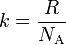The value of this constant k is approximately 1. 380650 x 10 -23 joules per Kelvin (J · K -1)or 1.380650 × 10−16 erg per Kelvin. Generally, the energy present in a gas molecule is directly proportional to the absolute temperature. So, if there is an increase in the temperature, the same increase takes place with respect to the kinetic energy per molecule. As a gas is heated, the molecules that it consists of move rapidly, producing an increase in pressure if the gas is contained in a space of constant volume or an increased volume if the pressure is constant.

The physical significance of this constant k is that it helps to calculate the amount of energy (heat) that corresponds to random thermal motions of a substance’s molecules. If we consider a classical system that is at equilibrium, at a temperature T, the average energy per degree of freedom can be measured as kT/2. For a simple example, we can consider a gas that contains N non-interacting atoms, with each atom equipped with three translational degrees of freedom, i.e., it can move in either of three directions, x, y, or z. In this situation, the gas’s total thermal energy can be arrived at 3NkT/2.

Applications of Boltzmann Constant

A bridge between macroscopic and microscopic physics

Boltzmann constant k acts as a bridge from macroscopic to microscopic physics. In the macroscopic aspect, the ideal gas law states that for an ideal gas, the product of pressure p and volume V is proportional to the product of the amount of substance n (in moles) and absolute temperature T:where R is the gas constant (8.314 472(15) J K−1 mol−1).

Introduction of the Boltzmann constant here, converts the ideal gas law into an equation that corresponds to the microscopic properties of molecules.where N is the number of molecules of gas.

Equipartition of Energy

In a thermodynamic system that exists at an absolute temperature T, the thermal energy contained by each microscopic degree of freedom in the system is on the order of magnitude of kT/2 (i. e., about 2.07×10−21 J, or 0.013 eV, at room temperature).

Simple Gas Thermodynamics

Monatomic ideal gases are equipped with three degrees of freedom per atom, which correspond to the three spatial directions, meaning that each atom possesses a thermal energy of 1.5kT. Generally, this energy can be represented as DkT/2, where D is equal to the number of spatial dimensions. This observation also corresponds well with experimental data. The thermal energy is useful in the calculation of the root mean square of the speed of the atoms, which is of inverse proportion to the square root of the atomic mass. At room temperature, the root mean square speeds calculated for gases such as helium (1370 m/s) and xenon (240 m/s) accurately reflect this calculation.

According to kinetic theory, the average pressure p for an ideal gas is: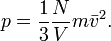Substituting the value of average translational kinetic energy,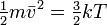gives,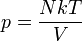leading to the reformation of the ideal gas equation.

The same ideal gas equation applies well for molecular gases, but in such cases, the form for heat capacity becomes more complicated as such molecules are equipped with new internal degrees of freedom, in addition to the three degrees of freedom for the entire molecule’s movement. For example, a diatomic atom possesses approximately five degrees of freedom per molecule.

Boltzmann Factors

A system in equilibrium at a temperature T, have a probability p of occupying a state i with energy e, which is weighted by the corresponding Boltzmann factor:where Z is the partition function. The important factor here again, is the energy-like quantity, kT. The consequences of this include the Arrhenius equation in chemical kinetics as well as the results for the ideal gases discussed earlier.

Statistical Definition of Entropy

In the field of statistical mechanics, entropy S of an isolated system at thermodynamic equilibrium is defined as the natural logarithm of W, which corresponds to the number of distinct microscopic states that are available to the system, taking into account the macroscopic constraints such as fixed total energy E.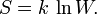This equation, relating the microscopic details/microstates of the system via W to its macroscopic state via the entropy S, is central to the field of statistical mechanics. Its incredible significance can be understood from the fact that it is the equation that is inscribed on Boltzmann’s tomb.

k, the constant of proportionality, helps make the statistical mechanical entropy equal to the classical thermodynamic entropy of Clausius: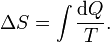A rescaled dimensionless entropy in microscopic terms can also be chosen instead: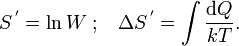This form is more natural and this rescaled entropy is an exact fit to Shannon’s information entropy. Here, the characteristic energy kT is the heat that is needed to increase the rescaled entropy by one nat.

Semiconductor Physics – Thermal Voltage

The relationship between the flow of electrical current and electrostatic potential in a semiconductor, across a p-n junction is dependent upon a characteristic voltage that is referred to as the thermal voltage, V. This thermal voltage V depends on absolute temperature T, as: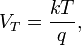where q denotes the magnitude of the electrical charge on the electron, with a value 1.602 176 487(40) ×10−19 C and k is the Boltzmann constant in Joules/K. The Boltzmann constant can be expressed in electron volts as 8.617 343(15) ×10−5 eV/K, aiding easy calculation at room temperature (≈ 300 K). The approximate value of the thermal voltage is 25.85 mill volts ≈ 26 mV. This voltage plays an important role in plasmas and electrolyte solutions, where it helps to determine how much the spatial distribution of electrons or ions is affected by a boundary held at a fixed voltage.

105 queries in 0.190 seconds.